### Home > CALC > Chapter 9 > Lesson 9.4.2 > Problem9-129

9-129.
1. Timmy is tired. He does not want to add all the infinite terms of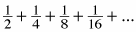So instead, he just adds the first three terms. Homework Help ✎1. How far is his result from the actual sum of the infinite series? (This is called his "error.")

2. What would his error have been if he added the first four terms?

3. Generalize his error. That is, if he adds up n terms of this geometric series, what will his error be?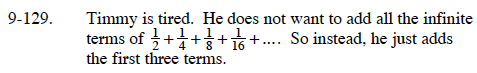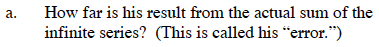For an infinite geometric series: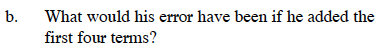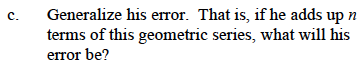For a finite geometric series:

Substitute a = 1/2 and r = 1/2 into the formulas in the hints then evaluate.